# 1566 How to Make a Magic Square Snowflake

### Today’s Puzzle:

Can you place all the numbers from one to nine in a 3 × 3 grid so that the numbers on each of the four edges and the numbers on each of the four lines of symmetry (where the fold lines are) have the same sum?Here are some hints to get you started:

Begin by grouping and adding the numbers in this fashion:
Odd numbers 1 + 9 = 10,
Even numbers 2 + 8 = 10.
Odd numbers 3 + 7 = 10,
Even numbers 4 + 6 = 10, leaving you with just
5, which has no partner so it must go in the center of the magic square.

Those four sums plus 5 will form the four lines of symmetry, each with a magic sum of 10 + 5 = 15. Where do you put the sums in the magic square? Notice that the only way the edges can equal an odd number like 15 is to add two even numbers and one odd. (Adding two odd numbers and an even will never give you an odd number.) That means even numbers must go in the corners and an odd number will go between them.

Place the 1 in the middle of any edge. Place the nine in the middle on the opposite edge. What numbers will go on the edge with the 1? They must be two numbers that add up to 15 – 1 = 14. Only two of the even numbers add up to 14. Place them on the square on either side of the 1. Place their partners from the sums to 10 that are written above on their same lines of symmetry. Now you only have two odd numbers left to place. Place them so that those edges add up to 15.

Using a hole punch to make the numbers instead of writing numerals makes the snowflake even better: There are eight different orientations for the numbers to be on the snowflake, but all eight ways really only form one possible snowflake.### How to Make Your Own Magic Square Snowflake:

You will need an 8 1/2 by 11 sheet of white printer paper, scissors, and a hole punch. Decorative scissors are optional.

1. Begin with an 8 1/2 by 11 sheet of white printer paper. Follow  Paula Krieg’s instructions on how to fold and cut it into an 8 1/2 × 8 1/2 perfect square.
2. Make a crease along both diagonals of your square. Unfold.3. Follow EZ Origami’s instructions on how to fold your square into thirds. Unfold.
4. Turn your square 90° and follow EZ Origami’s instructions again to divide your square into thirds in the other direction.5. Fold snowflake in half vertically and then horizontally. Unfold.6. If desired, use decorative scissors to give your snowflake a pretty edge. You can cut two edges at the same time. Unfold.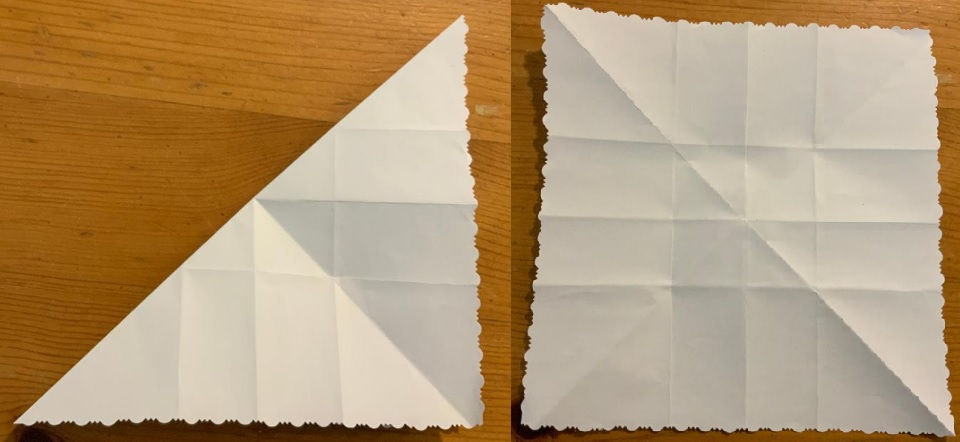7. Make a narrow cut along the folds of the smaller square edges, taking care not to cut where the folds intersect. Unfold. (Do not use decorative scissors for this step.)8. Use the grid with numerals that you made earlier and a hole punch to place the right number of holes in each of the nine squares. An ordinary hole punch with handles will work, but I used the decorative one pictured below.I used the following facts to make my holes with my hole punch in the appropriate squares. Look at the snowflake picture above if clarification is needed:
1. Make a single punch in the center of its square.
2(1) = 2. Fold paper on diagonal, make a single punch above the diagonal.
2(3(1/2)) = 3. Fold paper on diagonal, make three half punches on the diagonal. Unfold.
2(2) = 4. Fold paper on diagonal, make two punches above the diagonal. Unfold.
4(1 + 1/4) = 5. The center square has four smaller squares. Fold center square to make one small square with four thicknesses, make 1/4 punch at corner of folds in center of snowflake. Make a single punch in center of small, thick square. Unfold.
2(3) = 6. Fold paper on diagonal, make three punches above the diagonal. Unfold.
2(3 + 1/2) = 7. Fold paper on diagonal, make half punch on center of diagonal and three punches above the diagonal. Unfold.
2(4) = 8. Fold paper on diagonal, make four punches above the diagonal. Unfold.
2((3 + 3(1/2)) = 9. Fold paper on diagonal, make three half punches on diagonal and three punches above the diagonal. Unfold.### Finding the Prime Factorization of 1566:

Since this is my 1566th post, I’ll explain how I find its prime factorization using as few divisions as I possibly can.

I know that 1566 can be divided by 9 because 1 + 5 + 6 + 6 = 18, a multiple of 9.
1566 ÷ 9 = 174.

I know that 174 can be divided by 6 because it is even and 1 + 7 + 4 = 12, a multiple of 3.
174 ÷ 6 = 29.

I show my work here:Now all that’s left to do is put the prime factors in numerical order with exponents.

### Factors of 1566:

• 1566 is a composite number.
• Prime factorization: 1566 = 2 × 3 × 3 × 3 × 29, which can be written 1566 = 2 × 3³ × 29.
• 1566 has at least one exponent greater than 1 in its prime factorization so √1566 can be simplified. Taking the factor pair from the factor pair table below with the largest square number factor, we get √1566 = (√9)(√174) = 3√174.
• The exponents in the prime factorization are 1,3 and 1. Adding one to each exponent and multiplying we get (1 + 1)(3 + 1)(1 + 1) = 2 × 4 × 2 = 16. Therefore 1566 has exactly 16 factors.
• The factors of 1566 are outlined with their factor pair partners in the graphic below.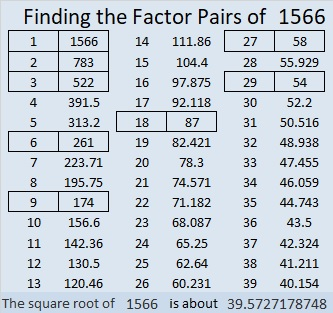### More about the Number 1566:

1566 is the hypotenuse of a Pythagorean triple:
1080-1134-1566 which is (20-21-29) times 54.

Since 1566 is a multiple of 3, it is the magic sum of this 3 × 3 magic square:That can happen for ANY multiple of 3. Multiples of 3 that are less than 15 must have negative numbers in their magic squares in order to be the magic sum. For example, zero is a multiple of 3 because 3 × 0 = 0. Here’s how zero is the magic sum of a magic square: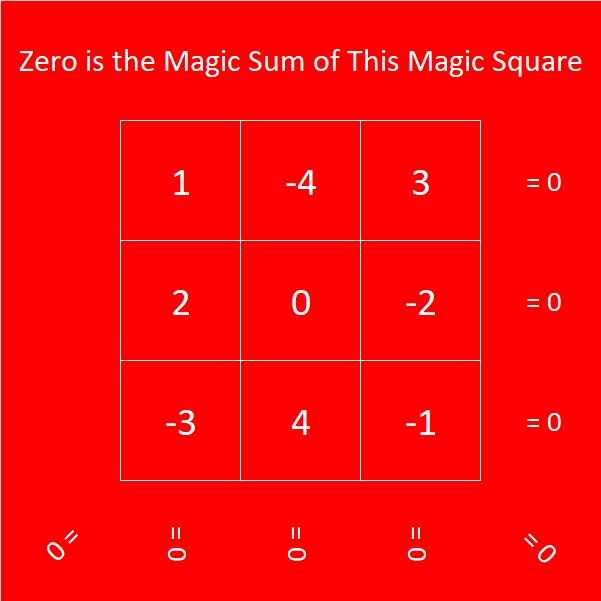# 1379 You Can Solve This Magic Square

I knew that 1379 was the magic sum of a 14 × 14 magic square because 14² = 196 and
(196)(197)/(2·14) = 1379.

I know how to solve a 4 × 4 magic square and when the dimensions of the magic square are odd numbers. I can also solve squares whose dimensions can be factored into any of those.  Clearly, the dimensions of a 14 × 14 magic square don’t qualify. I wondered if there was a simple way to solve it.I watched a video that explained how to use a 7 × 7 magic square to solve one that is
14 × 14
. You don’t have to watch the video to solve this magic square. Let me explain:

Notice that I’ve grouped the squares in the 14 × 14 magic square into 4 × 4 sub-grids. Now the magic square can behave more like a 7 × 7 magic square. Also, notice that the highest number in each sub-grid is 4 times the corresponding number in a 7 × 7 magic square.You should notice that many of the numbers appear in order along the diagonal of the 7  × 7 square. The trickiest part for me is always the upper right corner.

Unfortunately, the 4 × 4 sub-grids are not all the same. In the video, they were labeled X, Y, and Z. To make it simpler, I’ve color-coded them so that you can know how to place the four numbers in each sub-grid from lowest to highest.You can use this excel file,10 Factors 1373-1388 to first solve the 7 × 7 magic square and then use it and the three squares above to solve the 14 x 14 one. I would encourage you to give it a try! It is so satisfying to succeed!

Here’s a little more about the number 1379:

• 1379 is a composite number.
• Prime factorization: 1379 = 7 × 197
• 1379 has no exponents greater than 1 in its prime factorization, so √1379 cannot be simplified.
• The exponents in the prime factorization are 1, and 1. Adding one to each exponent and multiplying we get (1 + 1)(1 + 1) = 2 × 2 = 4. Therefore 1379 has exactly 4 factors.
• The factors of 1379 are outlined with their factor pair partners in the graphic below.1379 is the hypotenuse of a Pythagorean triple:
196-1365-1379 which is 7 times (28-195-197)

# (1346÷2)×3 = 2019 Magic or Square?

I was inspired to make a 3 × 3 Magic Square where every number is different but the numbers in each row, column and diagonal added together equal the same number, 54:
I made it by taking a regular 3 × 3 Magic Square and adding 13 to each of its numbers.

What inspired me to do that? This magical tweet of a palindromic Magic Square for the Year 2019:

Yeah, I know my magic square isn’t quite as impressive. It might be more square than it is magic. It’s also less impressive than this number 2019 spelled out using fifty-one
4 × 4 Magic Squares.

Maybe you will be more impressed by this magic square that has 2019 as its Magic Sum?I could make that magic sum because 2019 is divisible by 3. Why is 673 in the center? Because 2019÷3 = 673.

673 × 2 = 1346. I’m sharing these magic squares in this post I’ve numbered 1346. In case you haven’t figured it out (1346÷2)×3 = 2019. Happy New Year!

Here’s more about the number 1346:

• 1346 is a composite number.
• Prime factorization: 1346 = 2 × 673
• 1346 has no exponents greater than 1 in its prime factorization, so √1346 cannot be simplified.
• The exponents in the prime factorization are 1, and 1. Adding one to each exponent and multiplying we get (1 + 1)(1 + 1) = 2 × 2 = 4. Therefore 1346 has exactly 4 factors.
• The factors of 1346 are outlined with their factor pair partners in the graphic below.1346 is the hypotenuse of a Pythagorean triple:
770-1104-1346 which is 2 times 385-552-673

# Mathemagical Properties of 1105

1105 is the magic sum of a 13 × 13 magic square. Why?
Because 13×13 = 169 and 169×170÷2÷13 = 13×85 = 1105.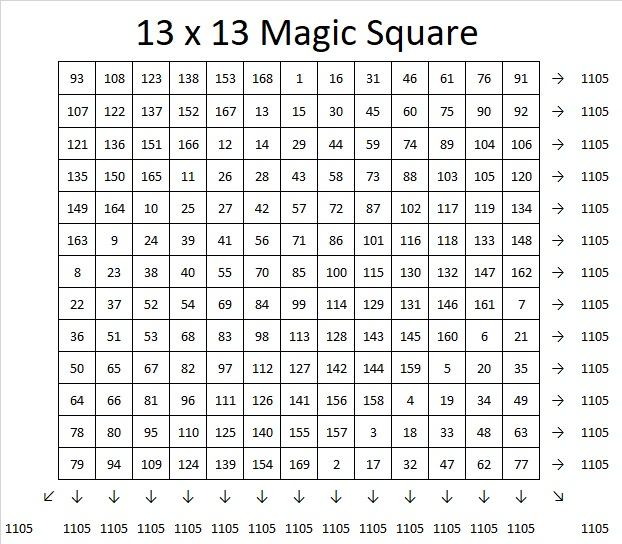If you follow the location of the numbers 1, 2, 3, 4, all the way to 169 in the magic square, you will see the pattern that I used to make that magic square. If you click on 10-factors-1102-1110  and go to the magic squares tab, you can use the same pattern or try another to create an 11 × 11, 13 × 13, or 15 × 15 magic square. The sums on the rows, columns, and diagonals will automatically populate as you write in the numbers so you can verify that you have indeed created a magic square.

1105 tiny squares can be made into a decagon so we say it is a decagonal number: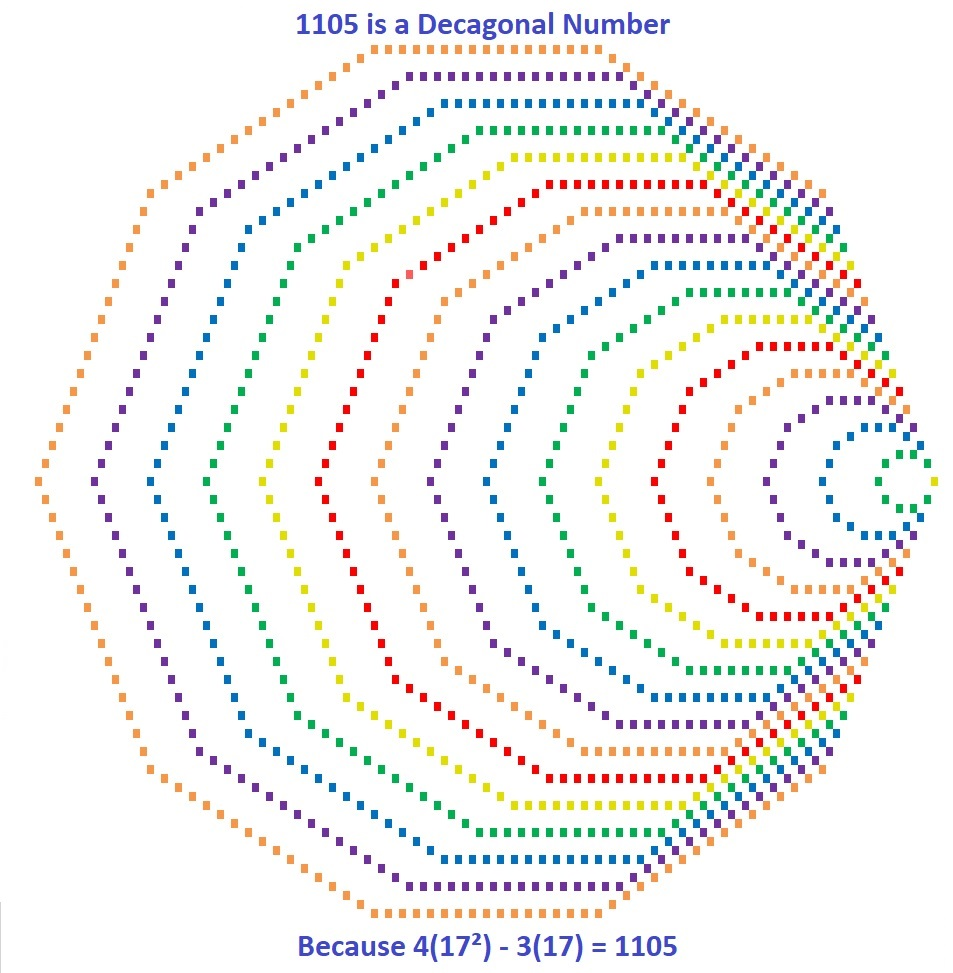Those 1105  tiny squares can also be arranged into a centered square: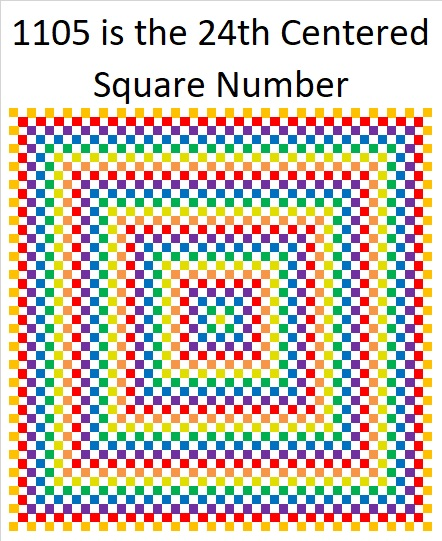Why is 1105 the 24th Centered Square Number? Because it is the sum of consecutive square numbers:
24² + 23² = 1105

But that’s not all! 1105 is the smallest number that is the sum of two squares FOUR different ways:

24² + 23² = 1105
31² + 12² = 1105
32² + 9² = 1105
33² + 4² = 1105

1105 is also the smallest number that is the hypotenuse of THIRTEEN different Pythagorean triples. Yes, THIRTEEN! (Seven was the most any previous number has had.) It is also the smallest number to have FOUR of its Pythagorean triplets be primitives (Those four are in blue type.):

47-1104-1105 calculated from 24² – 23², 2(24)(23), 24² + 23²
105-1100-1105 which is 5 times (21-220-221)
169-1092-1105 which is 13 times (13-84-85)
264-1073-1105 calculated from 2(33)(4), 33² – 4², 33² + 4²
272-1071-1105 which is 17 times (16-63-65)
425-1020-1105 which is (5-12-13) times 85
468-1001-1105 which is 13 times (36-77-85)
520-975-1105 which is (8-15-17) times 65
561-952-1105 which is 17 times (33-56-85)
576-943-1105 calculated from 2(32)(9), 32² – 9², 32² + 9²
663-884-1105 which is (3-4-5) times 221
700-855-1105 which is 5 times (140-171-221)
744-817-1105 calculated from 2(31)(12), 31² – 12², 31² + 12²

Why is it the hypotenuse more often than any previous number? Because of its factors! 1105 = 5 × 13 × 17, so it is the smallest number that is the product of THREE different Pythagorean hypotenuses.

It gets 1 triple for each of its three individual factors: 5, 13, 17, 2 triples for each of the three ways the factors can pair up with each other: 65, 85, 221, and four primitive triples for the one way they can all three be together: 1105. Thus it gets 2º×3 + 2¹×3 + 2²×1 = 3 + 6 + 4 = 13 triples.

Speaking of factors, let’s take a look at 1105’s factoring information:

• 1105 is a composite number.
• Prime factorization: 1105 = 5 × 13 × 17
• The exponents in the prime factorization are 1, 1, and 1. Adding one to each and multiplying we get (1 + 1)(1 + 1)(1 + 1) = 2 × 2 × 2 = 8. Therefore 1105 has exactly 8 factors.
• Factors of 1105: 1, 5, 13, 17, 65, 85, 221, 1105
• Factor pairs: 1105 = 1 × 1105, 5 × 221, 13 × 85, or 17 × 65
• 1105 has no square factors that allow its square root to be simplified. √1105 ≈ 33.24154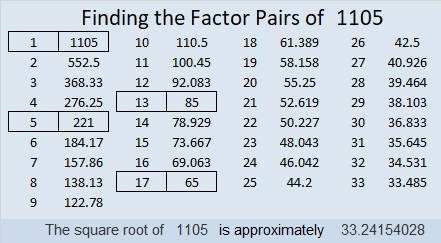1105 is also a palindrome in four different bases, and I also like the way it looks in base 8:
It’s 10001010001 in BASE 2 because 2¹º + 2⁶ + 2⁴ + 2º = 1105,
101101 in BASE 4 because 4⁵ + 4³ + 4² + 4º = 1105,
2121 in BASE 8 because 2(8³) + 1(8²) + 2(8) + 1(1) = 1105,
313 in BASE 19 because 3(19²) + 1(19) + 3(1) = 1105
1M1 in BASE 24 (M is 22 base 10) because 24² + 22(24) + 1 = 1105

Last, but certainly not least, you wouldn’t think 1105 is a prime number, but it is a pseudoprime: the second smallest Carmichael number. Only Carmichael number 561 is smaller than it is.

A Carmichael number is a composite number that behaves like a prime number by giving a false positive to all of certain quick prime number tests:
1105 passes the test p¹¹⁰⁵ Mod 1105 = p for all prime numbers p < 1105. Here is an image of my computer calculator showing 1105 passing the first five tests! Only a prime number should pass all these tests.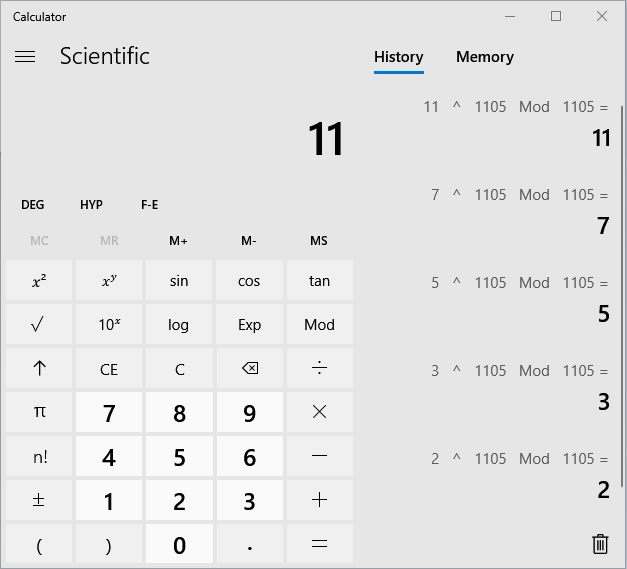1105 is indeed a number with amazing mathemagical properties!

# 880 Flip the Diagonals!

There are 880 different 4 × 4 Magic Squares. (In addition, each of those can be rotated and/or reflected to make 880 × 8 Magic Squares.) I have found a few that start with some simple ways to order the numbers 1 to 16 in the boxes and then flip the diagonals to create a perfect Magic Square.

For this first one I began by putting the numbers 1-4 in one box with the numbers 13-16 in the cater-corner box. Then 5-8 and 9-12 occupy the other spaces. Then I flipped the diagonals and got a Magic Square!If we flip the locations of the numbers 5-8 and the numbers 9-12, it still works:This third Magic Square begins with the most common way to order the numbers from 1 to 16:With just a little tweaking of that most common way to order the numbers, I was able to make the following three Magic Squares. Begin by switching the first two rows with each other and the last two rows with each other. Notice in every beginning square the diagonals already equal 34.We can also switch the first two rows with the last two rows, but that just gives us a reflection of the one just above it. (The colors don’t match, but it is still a reflection!)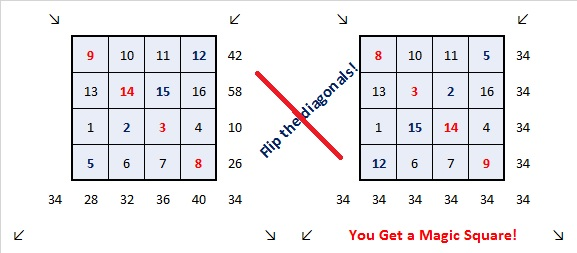Try switching the first two columns with the last two columns. You’ll get another different one.There are MANY more ways to do a 4 × 4 Magic Square.

Last week’s excel file, 12 factors 864-874, included some Magic Square templates including the 4 × 4 one. You can use that file to find more Magic Squares. Go ahead give it a try!

Here’s a little more about the number 880:

880 is the hypotenuse of a Pythagorean triple:

• 528-704-880, which is (3-4-5) times 176.

880 is the sum of the twelve prime numbers from 47 to 101.

• 880 is a composite number.
• Prime factorization: 880 = 2 × 2 × 2 × 2 × 5 × 11, which can be written 880 = 2⁴ × 5 × 11
• The exponents in the prime factorization are 4, 1 and 1. Adding one to each and multiplying we get (4 + 1)(1 + 1)(1 + 1) = 5 × 2 × 2 = 20. Therefore 880 has exactly 20 factors.
• Factors of 880: 1, 2, 4, 5, 8, 10, 11, 16, 20, 22, 40, 44, 55, 80, 88, 110, 176, 220, 440, 880
• Factor pairs: 880 = 1 × 880, 2 × 440, 4 × 220, 5 × 176, 8 × 110, 10 × 88, 11 × 80, 16 × 55, 20 × 44 or 22 × 40
• Taking the factor pair with the largest square number factor, we get √880 = (√16)(√55) = 4√55 ≈ 29.66479.# 870 If You Can Solve a 3×3 and a 4×4 Magic Square, Then You Can Solve a 12×12 Magic Square

It’s simple multiplication, folks!

3×4 = 12, so if you can solve a 3×3 Magic Square and a 4×4 Magic Square, then you can solve a 12×12 Magic Square, too.

Seriously. Really. It’s true! You really can solve a 12×12 Magic Square. I did it. Twice. You’ll see. You can do it, too.That solution consists of sixteen 3×3 Magic Squares that use all the numbers from 1 to 144. The one in the corner is the most familiar one. The other ones are just that familiar Magic Square plus 9, 18, 27 and a few other multiples of 9. I used the 4×4 Magic Square below as a guide as I placed the sixteen 3×3 Magic Squares on the excel file I made, 12 factors 864-874.make science GIFs like this at MakeaGif

There is 1 solution times 8 orientations (right-side-up, upside-down, etc.) for the 3×3 Magic Square, and there are 880 solutions times 8 orientations for the 4×4 Magic Square. That’s a lot of different possible solutions!

I also solved the 12×12 Magic Square by using nine 4×4 Magic Squares and placing them on the second grid in 12 factors 864-874. This arrangement also has many more possibilities than the one shown here.You can easily solve your own 12×12 Magic Square by opening this excel file, 12 factors 864-874, going to the 12×12 Magic Square tab, and following the directions.

Here’s more about the awesome number 870:

29 × 30 = 870. That means 870 is 29 × (29+1), so 870 is the sum of the first 29 even numbers.

870 is the sum of the ten prime numbers from 67 to 107.

Because 5 and 29 are its factors, 870 is the hypotenuse of four Pythagorean triples: ( Note that 5 × 29 = 145)

• 102-864-870, which is 6 times (17-144-145)
• 144-858-870, which is 6 times (24-143-145)
• 522-696-870, which is (3-4-5) times 174
• 600-630-870, which is (20-21-29) times 30

870 has some interesting representations in other bases:

• 606 in BASE 12, because 6(12²) + 0(12¹) + 6(12º) = 6(145) = 870
• 303 in BASE 17, because 3(17²) + 0(17¹) + 3(17º) = 3(290) = 870
• 110 in BASE 29, because 1(29²) + 1(29¹) + 0(29º) = 870
• T0 in BASE 30 (T is 29 base 10), because 29(30¹) + 0(30º) = 29(30) = 870

8 + 0 + 7 + 8³ + 0³ + 7³ = 870. Thank you OEIS.org for that fun fact.

• 870 is a composite number.
• Prime factorization: 870 = 2 × 3 × 5 × 29
• The exponents in the prime factorization are 1, 1, 1, and 1. Adding one to each and multiplying we get (1 + 1)(1 + 1)(1 + 1)(1 + 1) = 2 × 2 × 2 × 2 = 16. Therefore 870 has exactly 16 factors.
• Factors of 870: 1, 2, 3, 5, 6, 10, 15, 29, 30, 58, 87, 145, 174, 290, 435, 870
• Factor pairs: 870 = 1 × 870, 2 × 435, 3 × 290, 5 × 174, 6 × 145, 10 × 87, 15 × 58, or 29 × 30
• 870 has no square factors that allow its square root to be simplified. √870 ≈ 29.495762# 22 Factor Trees

22 is a composite number. 22 = 1 x 22 or 2 x 11. Factors of 22: 1, 2, 11, 22. Prime factorization: 22 = 2 x 11.When 22 is a clue in the FIND THE FACTORS 1 – 12 puzzles, use 2 and 11 as the factors.

‘Tis the season to use factor trees to find all the factors of a number. Here is a factor tree that shows all the prime factors of 560. Next to it is a factor tree for 324. Do you see all of their prime factors clearly?

Because sometimes one can’t “see the factors for the trees,” I recommend circling the prime factors or doing something else to make them more distinct.  Here are the trees again with every prime factor clearly visible:

I make logic puzzles based on the multiplication table. The puzzles for today’s post could also be called factor trees because you have to factor the clues to find the solution, and the puzzles are shaped like evergreen trees in whole or in part. I’ve even included a tree hanging from the ceiling, of all places! It may seem like a modern novelty, but people have been hanging Christmas trees upside down in Eastern Europe for centuries. To solve the puzzles either cut and paste the puzzles into a document to print or click 12 Factors 2013-12-12.